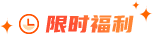### 大数据之两数相加题目描述

给出两个 非空 的链表用来表示两个非负的整数。其中，它们各自的位数是按照 逆序 的方式存储的，并且它们的每个节点只能存储 一位 数字。

如果，我们将这两个数相加起来，则会返回一个新的链表来表示它们的和。

您可以假设除了数字 0 之外，这两个数都不会以 0 开头。

示例：

输入：(2 -> 4 -> 3) + (5 -> 6 -> 4)

输出：7 -> 0 -> 8

原因：342 + 465 = 807

题目解析

设立一个表示进位的变量carried，建立一个新链表，把输入的两个链表从头往后同时处理，每两个相加，将结果加上carried后的值作为一个新节点到新链表后面。

代码实现

class Solution {

public ListNode addTwoNumbers(ListNode l1. ListNode l2) {

int carry = 0;

while(l1 != null || l2 != null)

{

int sum = carry;

if(l1 != null)

{

sum += l1.val;

l1 = l1.next;

}

if(l2 != null)

{

sum += l2.val;

l2 = l2.next;

}

// 创建新节点

carry = sum / 10;

cur.next = new ListNode(sum % 10);

cur = cur.next;

}

if (carry > 0) {

cur.next = new ListNode(carry);

}

}

}### 大数据师资团队更多>>

• 北京校区
• 大连校区
• 广州校区
• 成都校区
• 杭州校区
• 长沙校区
• 合肥校区
• 南京校区
• 上海校区
• 深圳校区
• 武汉校区
• 郑州校区
• 西安校区
• 青岛校区
• 重庆校区
• 太原校区
• 沈阳校区
• 南昌校区
• 哈尔滨校区